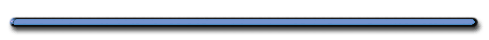﻿AP Physics

Gravity Near the Earth's Surface

NY - 9.803 m/s2

Denver - 9.796 m/s2

North Pole - 9.832 m/s2

 mg = GmmE r2g = GmE r2

G = Gravitational constant = 6.67 x 10-11 Nm2/kg2

Ex) Mars has a mass 1/10 that of Earth and a diameter 1/2 that of Earth.  The acceleration of a falling body near the surface of Mars is most nearly

 g = GmE r2

Substitute changes into equation

Giancoli p.  131) 32, 30

``
```Earth - mass
5.974 x 10²⁴ kg```
``

6,371 km

Ex) Prove that the orbital velocity of satellite does not depend on the mass

 mv2 = GmmE r r2

 v = (GmE/r)-1

From we equation we see that orbital V and Period T is only determined by orbital radius

The smaller the

the greater
the orbital velocity and

smaller period T

Astronauts in Space Station
are NOT weightless!!!Enrichmentwww.wpclipart.comThe Elegant Universe - PBSMythbusters Moon Landing Hoax 4 HQ